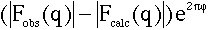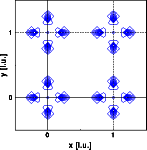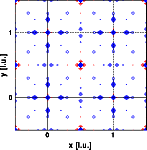Interactive Tutorial about Diffraction Structure solving: Part IIIStructure solution Tasks part 1 Answers part 1 Tasks part 2 Answers part 2 Tasks part 3 Answers part 3 Goto Contents If we assume that the structure is only partially solved, i.e. we know only the metal positions, we can calculate phase angles from this partial structures. These phase angles can be used to calculate the difference Fourier. The Fourier coefficients are now:Here PHI is the phase angle calculated from the partial structure solution. Applied to our example we obtain the following densities: (left image: X-ray difference density, right image neutron difference density)And again some homework before you go to the next chapter: The X-ray difference map shows only the oxygen positions, the neutron difference map shows significant differences at the metal positions as well. Why? The metal positions are the correct positions! © Th. Proffen and R.B. Neder, 2003